Test: Vector Analysis

# Test: Vector Analysis - Electrical Engineering (EE)

Test Description

## 20 Questions MCQ Test GATE Electrical Engineering (EE) 2023 Mock Test Series - Test: Vector Analysis

Test: Vector Analysis for Electrical Engineering (EE) 2023 is part of GATE Electrical Engineering (EE) 2023 Mock Test Series preparation. The Test: Vector Analysis questions and answers have been prepared according to the Electrical Engineering (EE) exam syllabus.The Test: Vector Analysis MCQs are made for Electrical Engineering (EE) 2023 Exam. Find important definitions, questions, notes, meanings, examples, exercises, MCQs and online tests for Test: Vector Analysis below.
Solutions of Test: Vector Analysis questions in English are available as part of our GATE Electrical Engineering (EE) 2023 Mock Test Series for Electrical Engineering (EE) & Test: Vector Analysis solutions in Hindi for GATE Electrical Engineering (EE) 2023 Mock Test Series course. Download more important topics, notes, lectures and mock test series for Electrical Engineering (EE) Exam by signing up for free. Attempt Test: Vector Analysis | 20 questions in 60 minutes | Mock test for Electrical Engineering (EE) preparation | Free important questions MCQ to study GATE Electrical Engineering (EE) 2023 Mock Test Series for Electrical Engineering (EE) Exam | Download free PDF with solutions
 1 Crore+ students have signed up on EduRev. Have you?
Test: Vector Analysis - Question 1

### Divergence and Curl of a vector field are _________.

Detailed Solution for Test: Vector Analysis - Question 1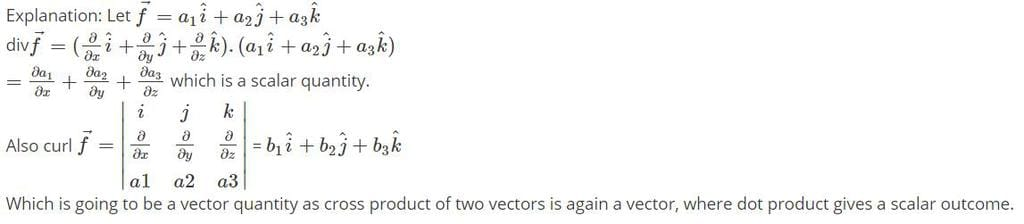Test: Vector Analysis - Question 2

### The two vector are RAM = 20ux + 18uy - 10uz and RAN = -10ux + 8uy + 15uz. The u of the triangle that bisects the interior angle at A is:

Detailed Solution for Test: Vector Analysis - Question 2

The non-unit vector in the required direction is: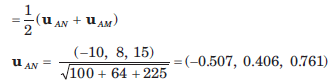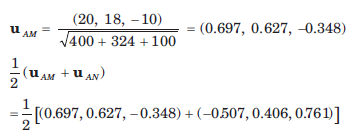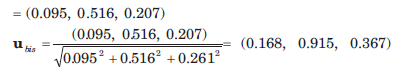Test: Vector Analysis - Question 3

### Two points in cylindrical coordinates are A(ρ = 5, Ø = 700 , z = -3) and B(ρ = 2, Ø = 300 , z = 1) A unit vector at A towards B is:

Detailed Solution for Test: Vector Analysis - Question 3

In cartesian coordinates: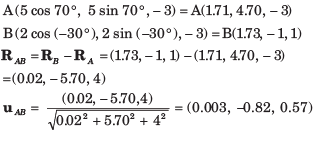Test: Vector Analysis - Question 4

The surface ρ = 2, ρ = 4,  Ø = 1000  ,Ø = 1300 ,z = 3  and z = 4.5 define a closed surface. The enclosed volume is:

Detailed Solution for Test: Vector Analysis - Question 4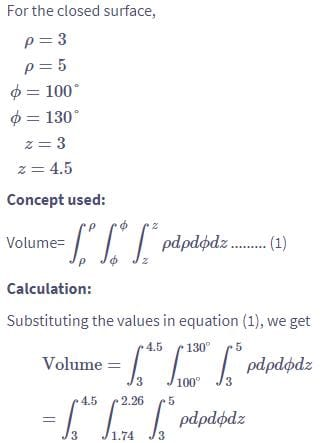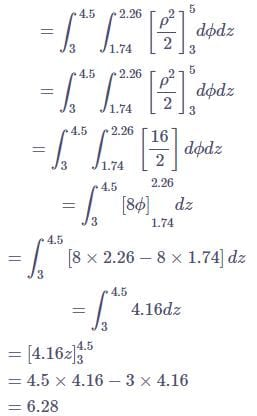Test: Vector Analysis - Question 5

The surface ρ = 2, ρ = 4,  Ø = 450  ,Ø = 1350 , z = 3  and z = 4 define a closed surface. The total area of the enclosing surface is:

Detailed Solution for Test: Vector Analysis - Question 5

Area is: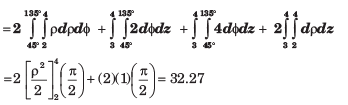Test: Vector Analysis - Question 6

The surface ρ = 3, ρ = 5,  Ø = 1000  ,Ø = 1300 , z = 3  and z = 4.5 define a closed surface. The length of the longest straight line that lies entirely within the volume is:

Detailed Solution for Test: Vector Analysis - Question 6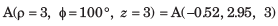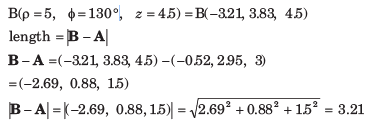Test: Vector Analysis - Question 7

A vector field H is: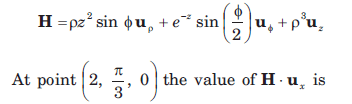Detailed Solution for Test: Vector Analysis - Question 7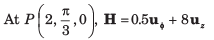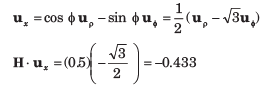Test: Vector Analysis - Question 8

A vector is A = yux +(x+z)uy  .At point P(-2, 6, 3)  A in cylindrical coordinate is:

Detailed Solution for Test: Vector Analysis - Question 8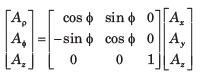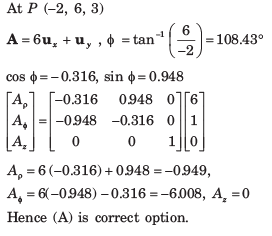Test: Vector Analysis - Question 9

The directional derivative of function φ = xy + yz + zx at point P(3, -3, -3) in the directiontoward point Q(4, -1, -1) is:

Detailed Solution for Test: Vector Analysis - Question 9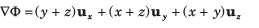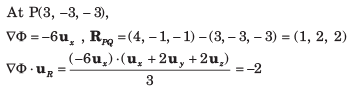Test: Vector Analysis - Question 10

The temperature in a auditorium is given by T = 2x2 +y2 - 2z2 . A mosquito located at (2, 2, 1) in the auditorium desires to fly in such a direction that it will get warm as soon as possible. The direction, in that it must fly is:

Detailed Solution for Test: Vector Analysis - Question 10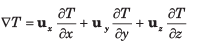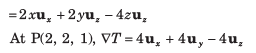Test: Vector Analysis - Question 11

The angle between the normal to the surface x2y + z = 3 and x ln z - y2 = -4 at the point of intersection (-1, 2, 1) is:

Detailed Solution for Test: Vector Analysis - Question 11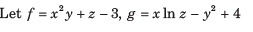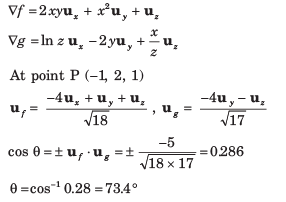Test: Vector Analysis - Question 12

The divergence of vector A = yzux + 4xyuy + yuz at point P(1, -2, 3) is:

Detailed Solution for Test: Vector Analysis - Question 12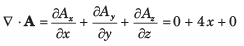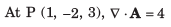Test: Vector Analysis - Question 13

The divergence of the vector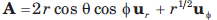at point P(1, 300 , 600 ) is:

Detailed Solution for Test: Vector Analysis - Question 13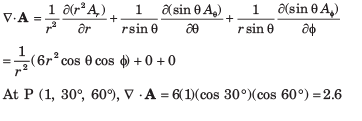Test: Vector Analysis - Question 14

Which of the quantities is nearest to the value of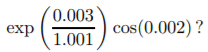Test: Vector Analysis - Question 15

The flux of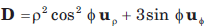over the closed surface of the cylinder 0 ≤ z ≤ 3 , ρ = 3 is:

Detailed Solution for Test: Vector Analysis - Question 15

By divergence theorem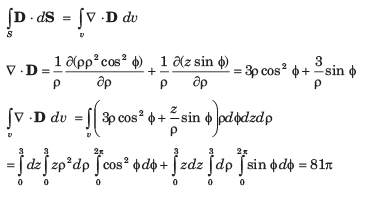Test: Vector Analysis - Question 16

The curl of vector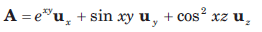is:

Detailed Solution for Test: Vector Analysis - Question 16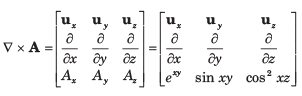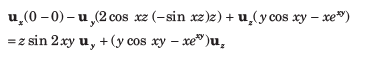Test: Vector Analysis - Question 17

The curl of vector field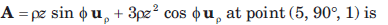Detailed Solution for Test: Vector Analysis - Question 17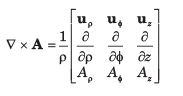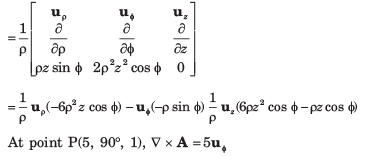Test: Vector Analysis - Question 18

The curl of vector field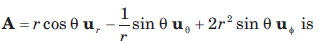Detailed Solution for Test: Vector Analysis - Question 18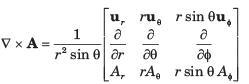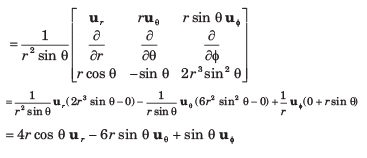Test: Vector Analysis - Question 19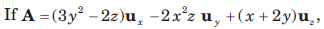the value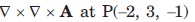is:

Detailed Solution for Test: Vector Analysis - Question 19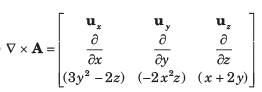Test: Vector Analysis - Question 20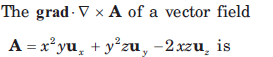Detailed Solution for Test: Vector Analysis - Question 20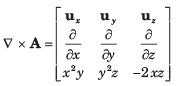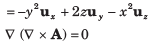## GATE Electrical Engineering (EE) 2023 Mock Test Series

22 docs|284 tests
Information about Test: Vector Analysis Page
In this test you can find the Exam questions for Test: Vector Analysis solved & explained in the simplest way possible. Besides giving Questions and answers for Test: Vector Analysis, EduRev gives you an ample number of Online tests for practice

## GATE Electrical Engineering (EE) 2023 Mock Test Series

22 docs|284 tests(Scan QR code)